0  447251  447259  447265  447269  447275  447277  447281  447287  447289  447295  447301  447305  447307  447311  447317  447319  447325  447329  447331  447335  447337  447341  447343  447345  447346  447347  447348

19.★(本小题满分10分)已知数列{an}、{bn}都是无穷等差数列,其中a1=3,b1=2,b2a2a3的等差中项,且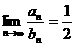.求极限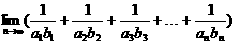的值.

∵2b2=a2+a3,即2(2+d2)=(3+d1)+(3+2d1),

∴2d2-3d1=2.①　　 2分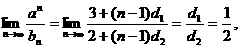d2=2d1,②　　　 4分

an=a1+(n-1)d1=3+(n-1)·2=2n+1,

bn=b1+(n-1)d2=2+(n-1)·4=4n-2.　　 6分10分

18.(本小题满分10分)已知数列{an}、{bn},其中an=1+3+5+…+(2n+1),bn=2n+4(n≥5),试问是否存在这样的自然数n,使得anbn成立？

an=1+3+5+…+(2n+1)=(n+1)2,

n=5时,a5=36,b5=25+4=36,此时a5=b5;

n=6时, a6=49,b6=26+4=68,此时a6<b6;

n=7时,a7=64,b7=27+4=132,此时a7<b7;

n=8时,a8=81,b8=28+4=260,此时a8<b8.

①当n=6时,显然不等式成立,∴n=6时,不等式an<bn成立;

②假设当n=k(k≥6)时,不等式成立,即ak<bk,也即(k+1)2<2k+4;当n=k+1时，bk+1=2k+1+4=2(2k+4)-4>2(k+1)2-4=2k2+4k-2,

∴当n=k+1时,不等式也成立.　　 8分

10分

17.(本小题满分8分)某校有教职工150人,为了丰富教工的课余生活,每天定时开放健身房和娱乐室.据调查统计,每次去健身房的人有10%下次去娱乐室,则在娱乐室的人有20%下次去健身房.请问,随着时间的推移,去健身房的人数能否趋于稳定?

an=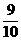an-1+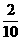bn-1=an-1+(150-an-1)=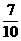an-1+30,

an=an-1+30.　　　　　　　　　 4分

an-100=(an-1-100).于是an-100=(a1-100)·()n-1,即an=100+()n-1·(a1-100).　 6分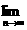an=100.故随着时间的推移,去健身房的人数稳定在100人左右.　　　　　　　 8分